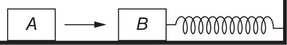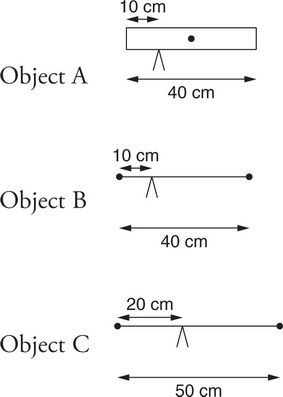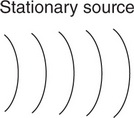# AP Physics 1 Practice Test 15

### Test Information10 questions18 minutes

1. Which of the following force diagrams could represent the forces acting on a block that slides to the right while slowing down?

2. Standing waves are produced by a 100-Hz generator in a string of fixed length. The tension in the string is increased until a new set of standing waves is produced. Will the wavelength of the new standing waves be greater than or less than the wavelength of the original standing waves?

3. Two electrically charged balls are separated by a short distance, producing a force of 50 μN between them. Keeping the charge of each ball the same, the mass of one of the balls but not the other is doubled. What is the new electric force between the balls?

4. A block of mass m is attached to a spring of force constant k. The mass is stretched a distance A from equilibrium and released from rest. At a distance x from the equilibrium position, which of the following represents the kinetic energy of the block?

5. A man stands with his hands to his sides on a frictionless platform that is rotating. Which of the following could change the angular momentum of the man-platform system?

1.Question below refers to the following information:

Block B is at rest on a smooth tabletop. It is attached to a long spring, which in turn is anchored to the wall. Identical block A slides toward and collides with block B. Consider two collisions, each of which occupies a duration of about 0.10 s:

Collision I: Block A bounces back off of block B.

Collision II: Block A sticks to block B.

In which collision, if either, is the period and frequency of the ensuing oscillations after the collision larger?

2. A string is fixed at one end but free to move at the other end. The lowest frequency at which this string will produce standing waves is 10 Hz. Which of the following diagrams represents how the string will look when it is vibrating with a frequency of 30 Hz?

3.Three equal-mass objects (A, B, and C) are each initially at rest horizontally on a pivot, as shown in the figure. Object A is a 40 cm long, uniform rod, pivoted 10 cm from its left edge. Object B consists of two heavy blocks connected by a very light rod. It is also 40 cm long and pivoted 10 cm from its left edge. Object C consists of two heavy blocks connected by a very light rod that is 50 cm long and pivoted 20 cm from its left edge. Which of the following correctly ranks the objects’ angular acceleration about the pivot point when they are released?

4.A stationary wave source emits waves at a constant frequency. As these waves move to the right, they are represented by the wave front diagram shown here. Sometime later, the wave source is moving at a constant speed to the right. Which of the following wave front diagrams could represent the propagation of the waves produced by the source?

5.An object of mass m is attached to an object of mass 3m by a rigid bar of negligible mass and length L. Initially, the smaller object is at rest directly above the larger object, as shown in the figure. How much work is necessary to flip the object 180°, such that the larger mass is at rest directly above the smaller mass?